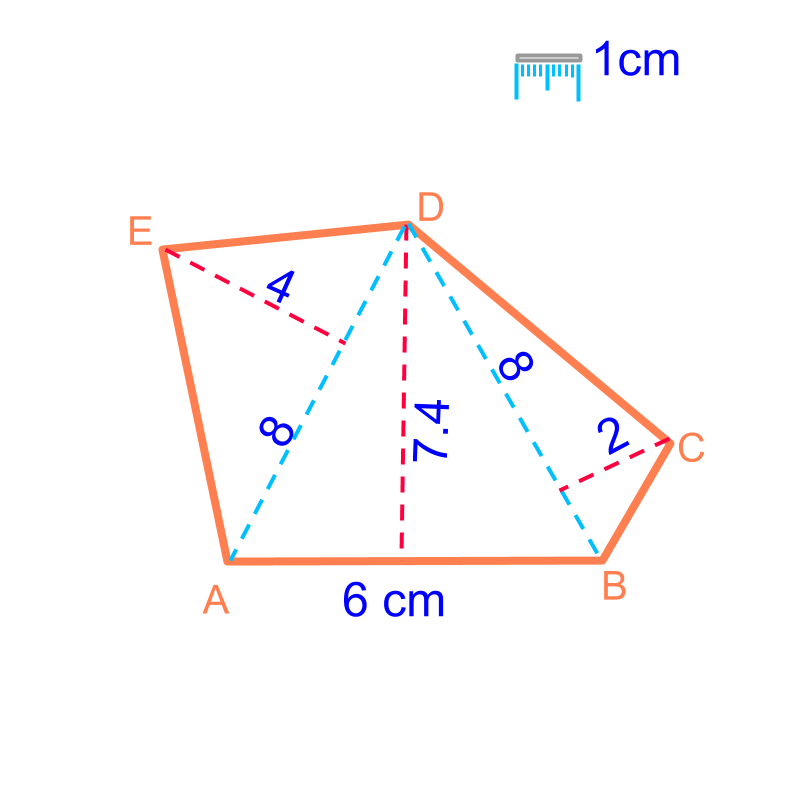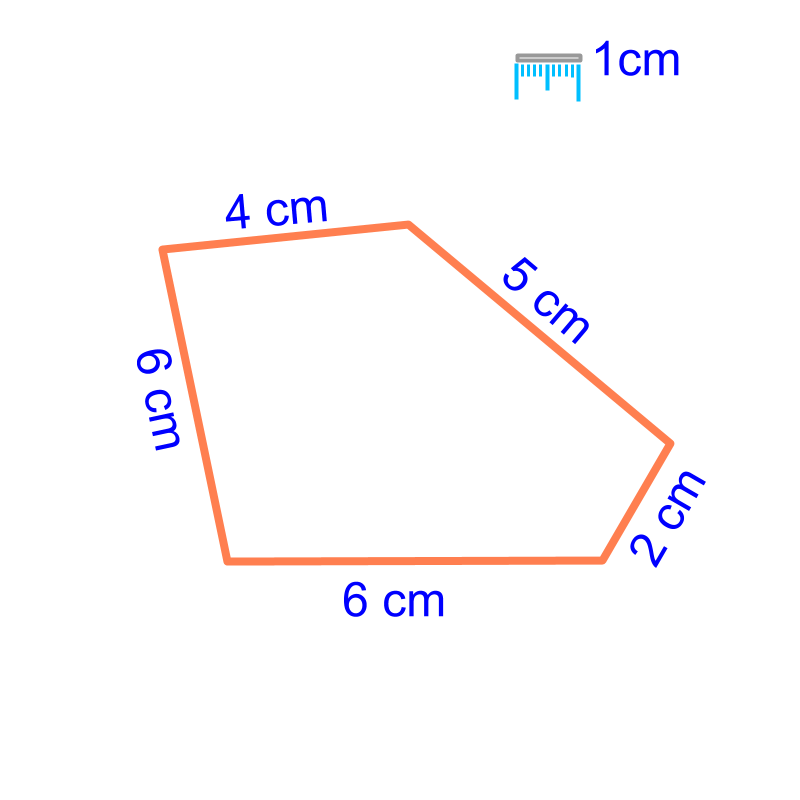maths > mensuration-basics

Area of Polygons

what you'll learn...

Overview

Area of a Polygon : Consider a polygon to be combination of known geometrical forms, mostly triangles.The geometrical forms and the formula for area are:

Area of a triangle $=\frac{1}{2}×\phantom{\rule{1ex}{0ex}}\text{base}\phantom{\rule{1ex}{0ex}}×\phantom{\rule{1ex}{0ex}}\text{height}$$= \frac{1}{2} \times \textrm{b a s e} \times \textrm{h e i g h t}$

Area of a trapezium $=\frac{1}{2}×\phantom{\rule{1ex}{0ex}}\text{sum of bases}\phantom{\rule{1ex}{0ex}}×\phantom{\rule{1ex}{0ex}}\text{height}$$= \frac{1}{2} \times \textrm{\sum o f b a s e s} \times \textrm{h e i g h t}$

Area of a parallelogram $=\phantom{\rule{1ex}{0ex}}\text{base}\phantom{\rule{1ex}{0ex}}×\phantom{\rule{1ex}{0ex}}\text{height}$$= \textrm{b a s e} \times \textrm{h e i g h t}$

Area of a kite $=\frac{1}{2}×\phantom{\rule{1ex}{0ex}}\text{major-diagonal}\phantom{\rule{1ex}{0ex}}×\phantom{\rule{1ex}{0ex}}\text{d2}$$= \frac{1}{2} \times \textrm{m a j \mathmr{and} - \mathrm{di} a g o n a l} \times \textrm{d 2}$

all are combination of trianglesConsider the polygon shown in the figure. To find the area of the polygon, "consider the polygon as combination of triangles".Area of the polygon

$=$$=$ area of $△ABD+$$\triangle A B D +$ area of $△DBC$$\triangle D B C$ + area of $△ADE$$\triangle A D E$.

The formula for area of triangle is used to find the area of the polygons.

What is the area of a quadrilateral with all internal angles ${90}^{\circ }$${90}^{\circ}$ and two sides $4$$4$ cm and $3$$3$cm?
This can be solved as
1. two triangles with combined area $2×\frac{1}{2}×4×3=12c{m}^{2}$$2 \times \frac{1}{2} \times 4 \times 3 = 12 c {m}^{2}$
2. area of the rectangle $4×3=12c{m}^{2}$$4 \times 3 = 12 c {m}^{2}$

summary

Area of a Polygon : Consider a polygon to be combination of known geometrical forms, mostly triangles.The geometrical forms and the formula for area are:

Area of a triangle $=\frac{1}{2}×\phantom{\rule{1ex}{0ex}}\text{base}\phantom{\rule{1ex}{0ex}}×\phantom{\rule{1ex}{0ex}}\text{height}$$= \frac{1}{2} \times \textrm{b a s e} \times \textrm{h e i g h t}$

Area of a trapezium $=\frac{1}{2}×\phantom{\rule{1ex}{0ex}}\text{sum of bases}\phantom{\rule{1ex}{0ex}}×\phantom{\rule{1ex}{0ex}}\text{height}$$= \frac{1}{2} \times \textrm{\sum o f b a s e s} \times \textrm{h e i g h t}$

Area of a parallelogram $=\phantom{\rule{1ex}{0ex}}\text{base}\phantom{\rule{1ex}{0ex}}×\phantom{\rule{1ex}{0ex}}\text{height}$$= \textrm{b a s e} \times \textrm{h e i g h t}$

Area of a kite $=\frac{1}{2}×\phantom{\rule{1ex}{0ex}}\text{major-diagonal}\phantom{\rule{1ex}{0ex}}×\phantom{\rule{1ex}{0ex}}\text{d2}$$= \frac{1}{2} \times \textrm{m a j \mathmr{and} - \mathrm{di} a g o n a l} \times \textrm{d 2}$

Outline

The outline of material to learn "Mensuration basics : Length, Area, & Volume" is as follows.

•  Measuring Basics

→   Introduction to Standards

→   Measuring Length

→   Accurate & Approximate Meaures

→   Measuring Area

→   Measuring Volume

→   Conversion between Units of Measure

•  2D shapes

→   Perimeter of Polygons

→   Area of Square & rectangle

→   Area of Triangle

→   Area of Polygons

→   Perimeter and area of a Circle

→   Perimeter & Area of Quadrilaterals

•  3D shapes

→   Surface Area of Cube, Cuboid, Cylinder

→   Volume of Cube, Cuboid, Cylinder